# Periodic and Non-Periodic Functions

 Problem 1 A student is using an ANN to map two non-periodic and one periodic function as shown. The ANN will try to map the three functions in the range from -10 to 10. Build an appropriate training set to learn these functions. Assign an appropriate number of training cases using uniformly distributed values for x in the range from -10 to 10. Create a Neural Lab New Project called MappingNonPer to perform the simulations (you may check the Performance.lab checkbox to save you some typing).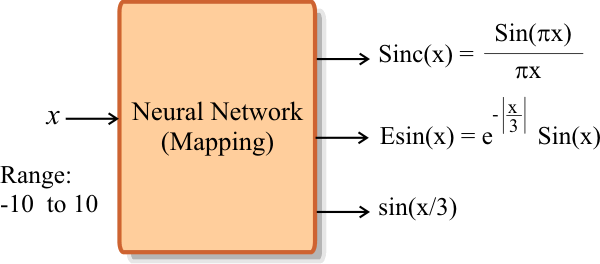Problem 2 Build a validation set for the functions using random values of x from -10 to 10. Use an appropriate number of validation cases.

 Tip The number of training cases depends directly on the complexity of the functions to map. The y = Sinc(x) and the y = Esin(x) are very complex functions when they are compared with y = sin(x) . Thus, the number of training cases must be adjusted to allow the network to see in detail the behavior of the functions.

 Tip A common mistake (when solving similar problems using ANNs) is to use the same parameters for the training set, the training and the ANN. The student must use common sense to adjust the different parameters to the specific problem.

 Tip The design of the training set involves deciding the number of rows (training cases) and the number of columns (number of samples). If a problem is not properly solved with a specific number of hidden neurons, it is recommended to increase the number of neurons in the hidden layers (occasionally, this will increase the number of required training cases). The proper of use of ANNs involves the adjustment of several parameters.

 Problem 3 Edit the Train.lab file to design and train an ANN for the functions of problem 1. Use one hidden layer.

 Problem 4 Edit the CheckTraining.lab file to check the training: (a) Compute the mean squared error for the ANN using the training set. (b) Plot the error for each training case. (c) Save the plot as a vector image (checkTraining.pdf and checkTraining.emf)

 Problem 5 In the network of problem 3, (a) modify the number of hidden neurons appropriately by creating the Performance.lab file. The code must plot the mse for the validation and for training as a function of the neurons in the hidden layer. This test may last several hours. (b) Save the plot as a vector image (performance.pdf and performance.emf)

 Problem 6 Edit the Validation.lab file to perform the validation of the ANN. (a) Compute the mean squared error for the ANN using the validation set. (b) Plot the error for each validation case (validation.pdf and validation.emf).

 Problem 7 Choose an appropriate number of neurons in the hidden layer and train the network. Write the file TrainSim.lab to compare the actual output of the network and the target using the training set.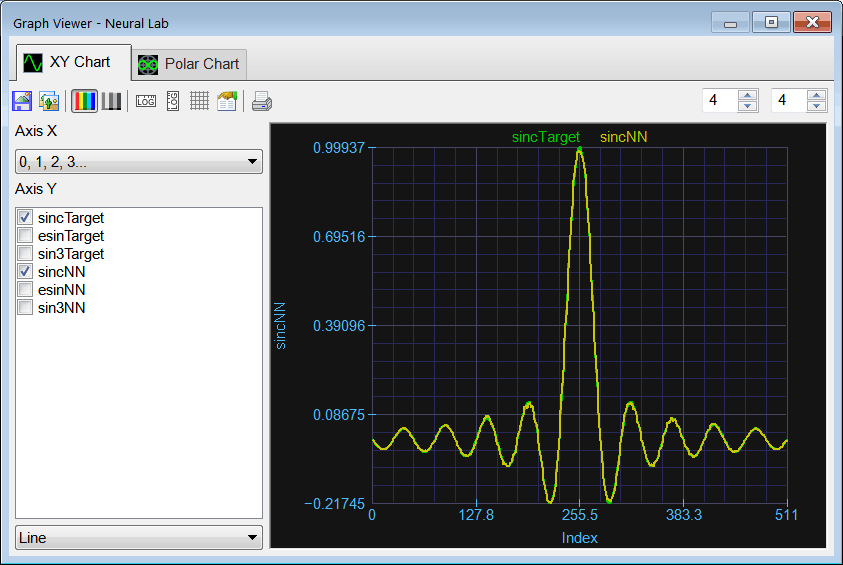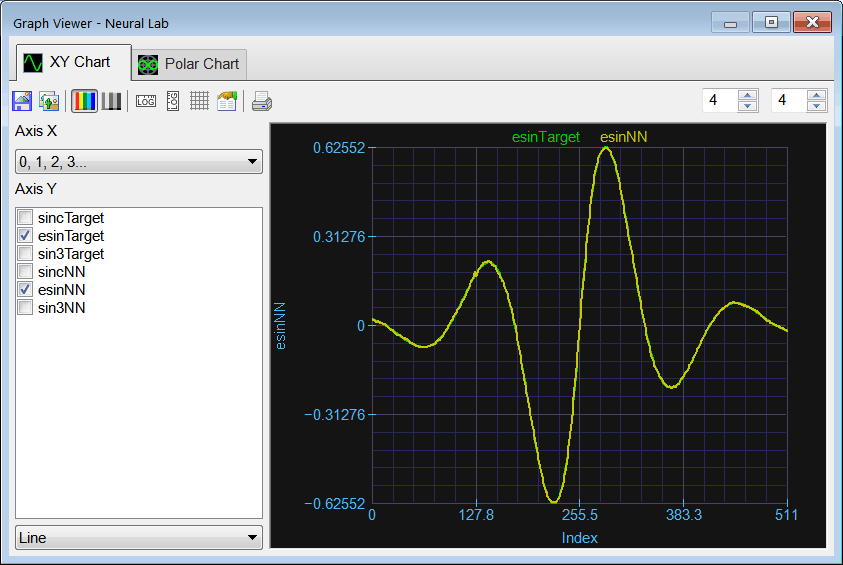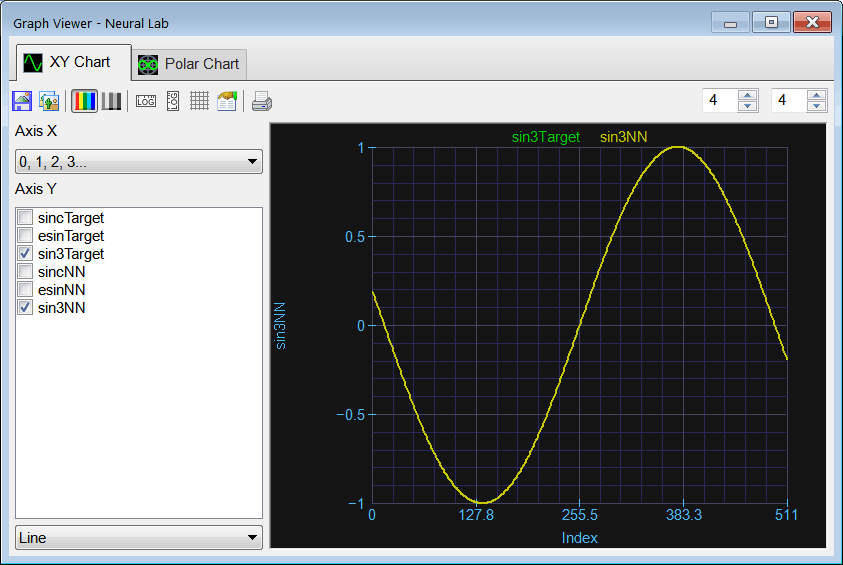Problem 8 Write the file ValidSim.lab to compare the actual output of the network and the target using the validation set. As the validation set has random input points, we cannot use the original validation set. Instead we will use a uniformly distributed validation set as shown (note that the number of points cannot be the same as the training set; in fact the number of points must be chosen carefully so that the cases in the validation set are NOT the same as those in the training set).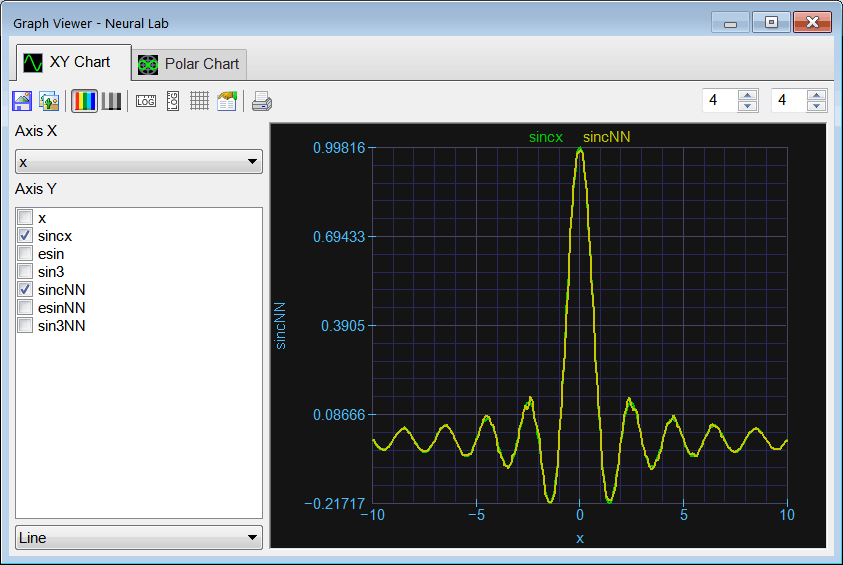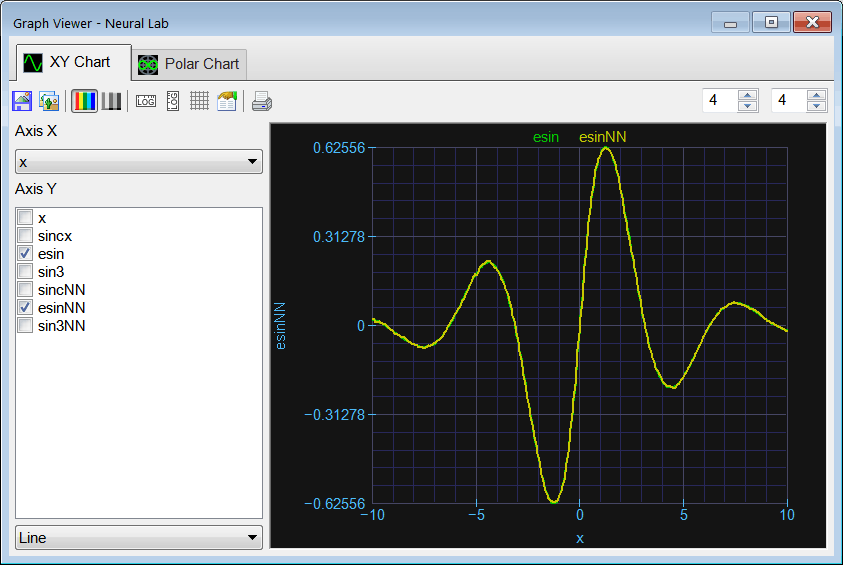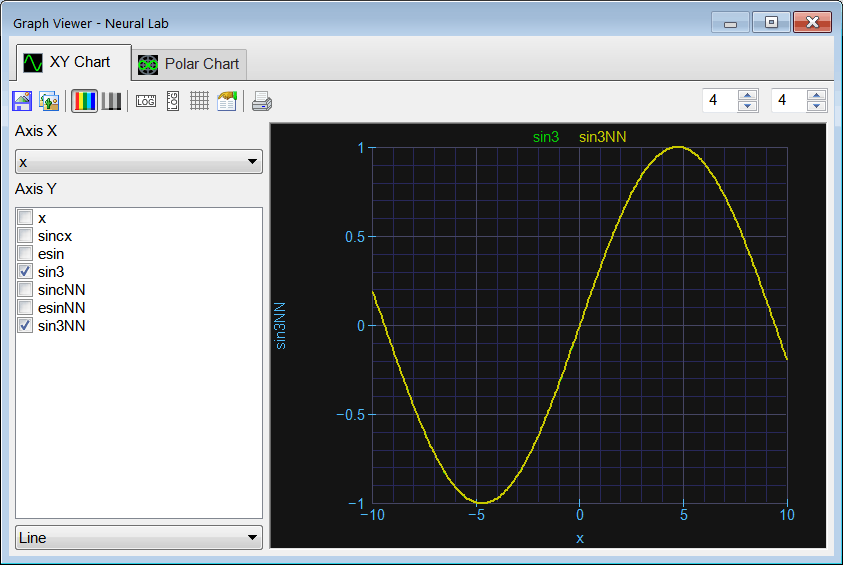Problem 9 Create an independent application for Microsoft Windows using C++ to compute the functions using direct computation and the ANN of problem 3.

 Problem 10 The student is not pleased with the accuracy (performance) of the network used in problem 3. What options does the student have to improve the network accuracy?

 Problem 11 Generate a report in Microsoft Word. Write some conclusions in the report focusing on the problems that were faced during the simulation and how these problems were or could be solved.